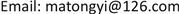﻿ 关于欧氏平面ℝ²中三角形的John定理 The John Theorem for Triangles in 2-Dimension Euclidean Space ℝ²

Vol. 08  No. 05 ( 2019 ), Article ID: 30417 , 7 pages
10.12677/AAM.2019.85109

The John Theorem for Triangles in 2-Dimension Euclidean Space ${ℝ}^{2}$

Tongyi Ma1, Tian Xiao2

1College of Mathematics and Statistics, Hexi University, Zhangye Gansu

2Class 1 of Grade 2016 of Information and Computation Science, Department of Mathematics, Hainan University, Haikou HainanReceived: May 5th, 2019; accepted: May 20th, 2019; published: May 27th, 2019ABSTRACT

In this paper, a description of the John contact points of a regular triangle was given. It was proved that the John ellipse of any triangle is circle if and only if this triangle is regular and that the John ellipse of a regular triangle is its inscribed circle.

Keywords:Triangle, John Theorem, John Ellipse, Barycentric Coordinates

1河西学院数学与统计学院，甘肃 张掖

2海南大学数学系信息与计算科学2016级1班，海南 海口1. 引言

$\underset{i=1}{\overset{m}{\sum }}{c}_{i}{u}_{i}\otimes {u}_{i}={I}_{n}$(1.1)

$\underset{i=1}{\overset{m}{\sum }}{c}_{i}{u}_{i}=0$(1.2)

${|x|}^{2}=\underset{i=1}{\overset{m}{\sum }}{c}_{i}{〈{u}_{i},x〉}^{2}$(1.3)

$x=\underset{i=1}{\overset{m}{\sum }}{c}_{i}〈x,{u}_{i}〉{u}_{i}$(1.4)

2. 准备知识

${S}_{1}:{S}_{2}:{S}_{3}={\mu }_{1}:{\mu }_{2}:{\mu }_{3}$

$\left(0:\frac{1}{2}:\frac{1}{2}\right),\left(\frac{1}{2}:0:\frac{1}{2}\right),\left(\frac{1}{2}:\frac{1}{2}:0\right)$

$\left(\begin{array}{ccc}0& \frac{1}{2}& \frac{1}{2}\\ \frac{1}{2}& 0& \frac{1}{2}\\ \frac{1}{2}& \frac{1}{2}& 0\end{array}\right)$(2.1)

3. 定理1的证明

$\underset{i=1}{\overset{3}{\sum }}{c}_{i}{B}_{i}=\frac{2}{3}\left(0:\frac{1}{2}:\frac{1}{2}\right)+\frac{2}{3}\left(\frac{1}{2}:0:\frac{1}{2}\right)+\frac{2}{3}\left(\frac{1}{2}:\frac{1}{2}:0\right)=\left(1:1:1\right)$

$x=\underset{i=1}{\overset{3}{\sum }}{c}_{i}〈x,{u}_{i}〉{u}_{i}$(3.1)

$\text{Span}\left({u}_{1},{u}_{2},{u}_{3}\right)={ℝ}^{2}$

$x={\alpha }_{1}{u}_{1}+{\alpha }_{2}{u}_{2}+{\alpha }_{3}{u}_{3}$

$\left\{\begin{array}{l}〈{u}_{1},x〉={\alpha }_{1}〈{u}_{1},{u}_{1}〉+{\alpha }_{2}〈{u}_{1},{u}_{2}〉+{\alpha }_{3}〈{u}_{1},{u}_{3}〉,\\ 〈{u}_{2},x〉={\alpha }_{1}〈{u}_{2},{u}_{1}〉+{\alpha }_{2}〈{u}_{2},{u}_{2}〉+{\alpha }_{3}〈{u}_{2},{u}_{3}〉,\\ 〈{u}_{3},x〉={\alpha }_{1}〈{u}_{3},{u}_{1}〉+{\alpha }_{2}〈{u}_{3},{u}_{2}〉+{\alpha }_{3}〈{u}_{3},{u}_{3}〉.\end{array}$

$G=G\left({u}_{1},{u}_{2},{u}_{3}\right)=\left(\begin{array}{ccc}〈{u}_{1},{u}_{1}〉& 〈{u}_{1},{u}_{2}〉& 〈{u}_{1},{u}_{3}〉\\ 〈{u}_{2},{u}_{1}〉& 〈{u}_{2},{u}_{2}〉& 〈{u}_{2},{u}_{3}〉\\ 〈{u}_{3},{u}_{1}〉& 〈{u}_{3},{u}_{2}〉& 〈{u}_{3},{u}_{3}〉\end{array}\right)$

$G{\alpha }^{\text{T}}={\beta }^{\text{T}}$(3.2)

$〈{u}_{i},{u}_{j}〉=-\mathrm{cos}\angle \left({a}_{i},{a}_{j}\right)$

$\mathrm{cos}\angle \left({a}_{i},{a}_{j}\right)=\frac{{a}_{ji}}{{a}_{j}}$

$\left(\begin{array}{ccc}1& -\frac{1}{2}& -\frac{1}{2}\\ -\frac{1}{2}& 1& -\frac{1}{2}\\ -\frac{1}{2}& -\frac{1}{2}& 1\end{array}\right)$

$\left\{\begin{array}{l}G{\alpha }^{\text{T}}={\beta }^{\text{T}},\\ \underset{i=1}{\overset{3}{\sum }}〈{u}_{i},x〉=0.\end{array}$

$\alpha =\left(\frac{2}{3}〈{u}_{1},x〉,\frac{2}{3}〈{u}_{2},x〉,\frac{2}{3}〈{u}_{3},x〉\right)$ 代入上面的方程组可知 $\alpha$ 是该方程组的一组解。因此 ${ℝ}^{2}$ 中的任意一点可以表示成(3.1)式的形式，定理1证毕。

4. 定理2的证明

$\underset{i=1}{\overset{m}{\sum }}{c}_{i}{u}_{i}\otimes {u}_{i}={I}_{n}$

${\int }_{{ℝ}^{n}}\underset{i=1}{\overset{m}{\prod }}{f}_{i}{\left(〈{u}_{i},x〉\right)}^{{c}_{i}}\text{d}x\le \underset{i=1}{\overset{m}{\prod }}{\left({\int }_{ℝ}{f}_{i}\left(t\right)\text{d}t\right)}^{{c}_{i}}$(4.1)

F. Barthe在文  中给出了引理4.1等式成立的一组必要条件，即下面的引理。

$\underset{i=1}{\overset{m}{\sum }}{c}_{i}{u}_{i}\otimes {u}_{i}={I}_{n}$

$m=n$

$\underset{i=1}{\overset{3}{\sum }}{c}_{i}{u}_{i}\otimes {u}_{i}={I}_{2}$(4.2)

$\underset{i=1}{\overset{3}{\sum }}{c}_{i}{u}_{i}=0$(4.3)

$K=\left\{x\in {ℝ}^{2}:〈x,{u}_{i}〉\le 1,1\le i\le 3\right\}$，则K也是 ${ℝ}^{2}$ 中的三角形。由于 ${\left\{{u}_{i}\right\}}_{1}^{3}$ 是A和B的接触点，所以

$A\subset \left\{x\in {ℝ}^{2}:〈x,{u}_{i}〉\le 1,1\le i\le 3\right\}=K$

$A=K$

${v}_{i}=\sqrt{\frac{2}{3}}\left(-{u}_{i},\frac{1}{\sqrt{2}}\right)\in {ℝ}^{3},i=1,2,3$

${d}_{i}=\frac{3}{2}{c}_{i},i=1,2,3$

$\underset{i=1}{\overset{3}{\sum }}{d}_{i}{v}_{i}\otimes {v}_{i}={I}_{3}$

${f}_{i}\left(t\right)=\left\{\begin{array}{l}{\text{e}}^{-t},\text{\hspace{0.17em}}\text{\hspace{0.17em}}如果t\ge 0,\\ 0,\text{\hspace{0.17em}}\text{\hspace{0.17em}}\text{\hspace{0.17em}}\text{\hspace{0.17em}}如果t<0.\end{array}$

$F\left(x\right)=\underset{i=1}{\overset{3}{\prod }}{f}_{i}{\left(〈{v}_{i},x〉\right)}^{{d}_{i}}$

${\int }_{{ℝ}^{3}}F\left(x\right)\le \underset{i=1}{\overset{3}{\prod }}{\left({\int }_{ℝ}{f}_{i}\left(t\right)\text{d}t\right)}^{{d}_{i}}=1$(4.4)

$〈{v}_{i},x〉=\frac{r}{\sqrt{3}}-\sqrt{\frac{2}{3}}〈{u}_{i},y〉,i=1,2,3$

$\begin{array}{c}F\left(x\right)=\mathrm{exp}\left[-\underset{i=1}{\overset{3}{\sum }}{d}_{i}\left(\frac{r}{\sqrt{3}}-\sqrt{\frac{2}{3}}〈{u}_{i},y〉\right)\right]\\ =\mathrm{exp}\left(-\sqrt{3}r+\sqrt{\frac{2}{3}}〈\underset{i=1}{\overset{3}{\sum }}{c}_{i}{u}_{i},y〉\right)\\ =\mathrm{exp}\left(-\sqrt{3}r\right)\end{array}$$S\left(K\right)\le 3\sqrt{3}$(4.5)

${v}_{i}=\sqrt{\frac{2}{3}}\left(-{u}_{i},\frac{1}{\sqrt{2}}\right)$

${v}_{j}=\sqrt{\frac{2}{3}}\left(-{u}_{j},\frac{1}{\sqrt{2}}\right)$

$0=〈{v}_{i},{v}_{j}〉=\frac{2}{3}〈{u}_{i},{u}_{j}〉+\frac{1}{2}$

The John Theorem for Triangles in 2-Dimension Euclidean Space ℝ²[J]. 应用数学进展, 2019, 08(05): 958-964. https://doi.org/10.12677/AAM.2019.85109

1. 1. Ball, K. (1992) Ellipsoids of Maximal Volume in Convex Bodies. Geometriae Dedicata, 41, 241-250. https://doi.org/10.1007/BF00182424

2. 2. Ball, K. (1997) An Elementary Introduction to Modern Convex Geom-etry. In: Levy, S., Ed., Flavors of Geometry, Cambridge University Press, New York, 1-58.

3. 3. Barthe, F. (1998) On a Reverse Form of the Brascamp-Lieb Inequality. Inventiones Mathematicae, 134, 335-361. https://doi.org/10.1007/s002220050267

4. 4. Brascamp, H.J. and Lieb, E.H. (1976) Best Contants in Young’s In-equality, Its Converse, and Its Generalization to More than Three Functions. Advances in Mathematics, 20, 151-173. https://doi.org/10.1016/0001-8708(76)90184-5

5. 5. Coxeter, H.S.M. (1969) Barycentric Coordinates. §13.7 in Introduction to Geometry, 2nd Edition, Wiley, New York, 216-221.

6. 6. John, F. (1948) Extremum Problems with Inequatlities as Subsidiary Conditions. Courant Anniversary Volume, Interscience, New York, 187-204.

7. 7. Lin, S., Ge, X. and Leng, G.-S. (2006) The John Theorem for Simplex. Journal of Shanghai University (English Edition), 10, 487-490. https://doi.org/10.1007/s11741-006-0043-4